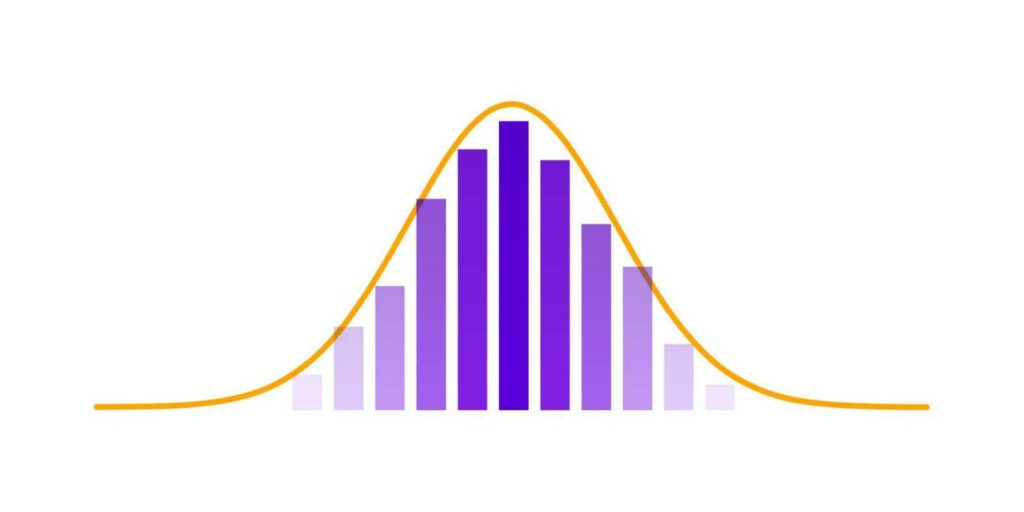The number of indicators that you can use when working with IQ Option is truly stunning. It is important to understand how certain technical analysis tools work in order to use them correctly. Today we are taking a closer look at a trend-following tool that can become a quite valuable addition to your trading system.

Ehler’s Fisher Transform, developed and introduced by J.F. Ehlers, is a popular technical analysis indicator that transforms the asset price into a Gaussian normal distribution. It may sound intimidating in the beginning but once you grasp the basic concept behind it, you will get a better understanding of how it works. This article will get a little bit more technical than usual. But don’t be afraid — all knowledge you get from it has a practical application and will help you improve your trading skills.

Read the full article to learn how Fisher Transform works and use it in trading. Note that this indicator works on all assets and timeframes.

Since we’ve mentioned a normal distribution, it would be wise to provide a basic explanation of this concept. A normal distribution (also called the bell curve) is a common probability distribution. For a normally distributed data set, the following will be true:

• the Mean value is equal to the Median value;
• the left and the right halves of the graph are symmetrical.

This is how it looks on a graph.A lot of things follow a normal distribution: heights of people, errors in measurements, blood pressure, marks on a test etc. However, there are also quantitative variables that do not follow a normal distribution, and the asset price is one of them. It makes them hard to predict and analyze.

That’s all for the theory. Now, let’s get to practice!

## How to use it in trading?

Ehler’s Fisher Transform transfigures prices into a normal distribution that can later be used in technical analysis. Since the indicator follows a normal distribution, extreme values of the indicator (both positive and negative) are quite uncommon. What does that mean for you? When Fisher Transform is above the zero line and goes up, the asset is overbought. When Fisher Transform is below the zero line and goes down, the asset is oversold. In both cases, the probability of a trend reversal gets higher with time. When receiving a buy or sell signal from another indicator, that confirms the signal sent by Fisher, traders consider opening a corresponding position.

Fisher Transform is usually compared to the Stochastic Oscillator. In fact, both work in a very similar fashion, demonstrating overbought and oversold positions, thus hinting at the moments when a price reversal can be expected. As with the Stochastic, when using Fisher Transform, you would want to use additional indicators to confirm its signals.

Remember that no technical analysis indicator is capable of providing accurate results 100% of the time. Fisher Transform is not an exception.